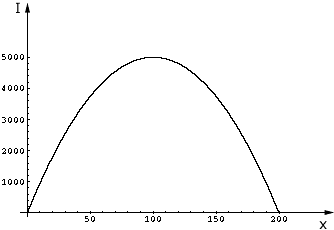Quandaries and Queries A company that sells x units of a product generates an income (I, in dollars) which is a function of x. The income generated is described by the equation I = (-1/2)x2 + 100x. Discuss how to determine the number of units that must be sold so that the company can maximize its income. What is the maximum income?   CONNIE PARENT SECONDARY Hi Connie, I am not sure what is expected but I would sketch a graph of the function I = (-1/2)x2 + 100x. You can solve (-1/2)x2 + 100x = 0 to see where the graph crosses the x-axis, and then either sketch a few points or use the fact that the graph is a parabola that opens downward to sketch the graph.From the graph you can see the value of x that maximizes the income. Penny Go to Math Central